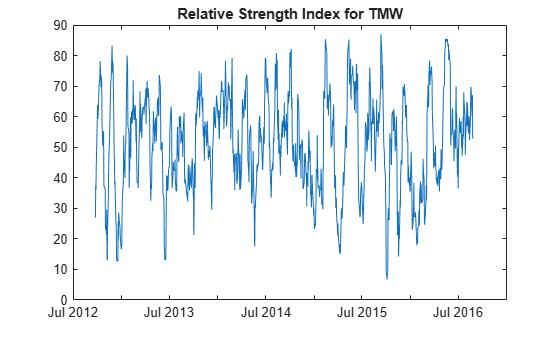# rsindex

Relative Strength Index (RSI)

Using a `fints` object for the `Data` argument of `rsindex` is not recommended. Use a matrix, `timetable`, or `table` instead for financial time series. For more information, see Convert Financial Time Series Objects fints to Timetables.

## Syntax

``index = rsindex(Data)``
``index = rsindex(___,Name,Value)``

## Description

example

````index = rsindex(Data)` calculates the Relative Strength Index (RSI) from the series of closing stock prices.```

example

````index = rsindex(___,Name,Value)` adds optional name-value pair arguments. ```

## Examples

collapse all

Load the file `SimulatedStock.mat`, which provides a timetable (`TMW`) for financial data for TMW stock.

```load SimulatedStock.mat index = rsindex(TMW); plot(index.Time,index.RelativeStrengthIndex) title('Relative Strength Index for TMW')```## Input Arguments

collapse all

Data with closing prices, specified as a matrix, table, or timetable. For matrix input, `Data` is `M`-by-`1` with closing prices. Timetables and tables with `M` rows must contain a variable named `'Close'` (case insensitive).

Data Types: `double` | `table` | `timetable`

### Name-Value Arguments

Specify optional comma-separated pairs of `Name,Value` arguments. `Name` is the argument name and `Value` is the corresponding value. `Name` must appear inside quotes. You can specify several name and value pair arguments in any order as `Name1,Value1,...,NameN,ValueN`.

Example: `index = rsindex(TMW,'WindowSize',10)`

Moving window size for relative strength index, specified as the comma-separated pair consisting of `'WindowSize'` and a scalar positive integer.

Data Types: `double`

## Output Arguments

collapse all

Relative strength index, returned with the same number of rows (`M`) and the same type (matrix, table, or timetable) as the input `Data`.

collapse all

### Relative Strength Index

Relative strength index is calculated by dividing the average of the gains by the average of the losses within a specified period.

`RS = (average gains) / (average losses)`

 Murphy, John J. Technical Analysis of the Futures Market. New York Institute of Finance, 1986, pp. 295–302.